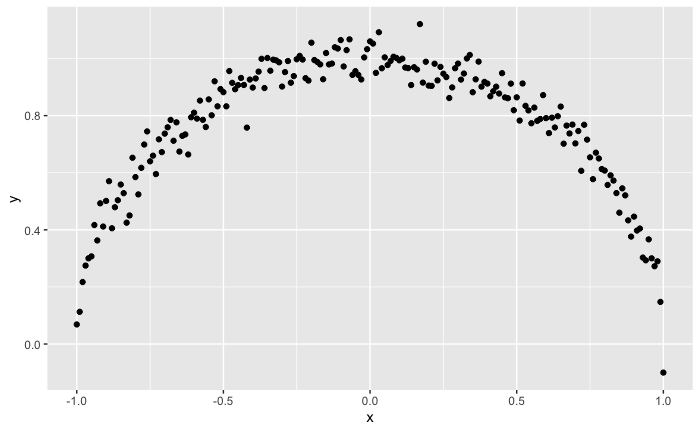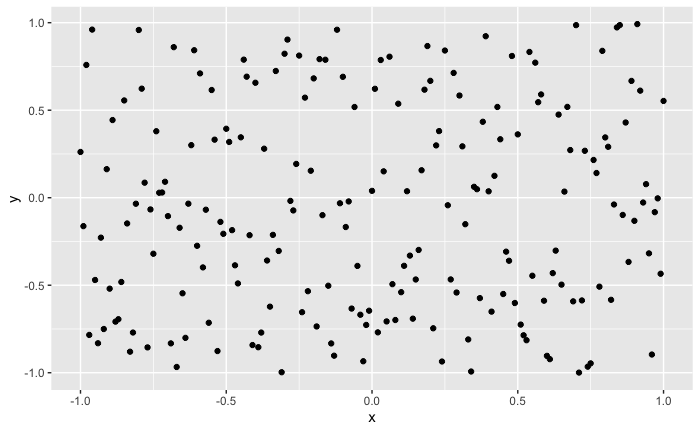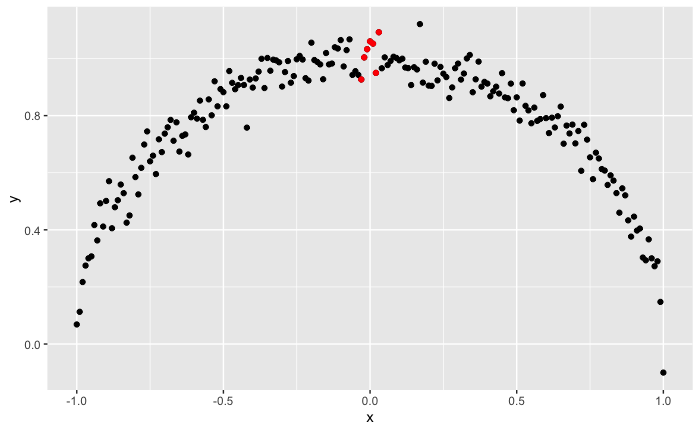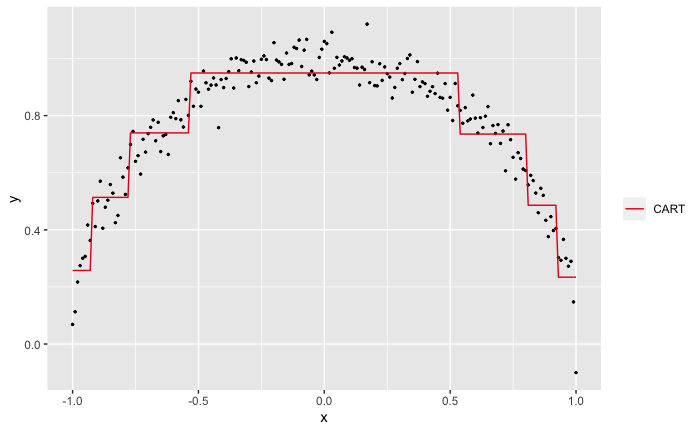### The Problem

When starting to work with a new dataset, it is useful to quickly pinpoint which pairs of variables appear to be strongly related. It helps you spot data issues, make better modeling decisions, and ultimately arrive at better answers.

The correlation coefficient is used widely for this purpose but it is well-known that it can’t detect non-linear relationships. Take a look at this scatterplot of two variables \(x\) and \(y\).

``````set.seed(42)
x <- seq(-1,1,0.01)
y <- sqrt(1 - x^2) + rnorm(length(x),mean = 0, sd = 0.05)

ggplot(mapping = aes(x, y)) +
geom_point()
``````It is obvious to the human eye that \(x\) and \(y\) have a strong relationship but the correlation coefficient between \(x\) and \(y\) is only -0.01.

Further, if either variable of the pair is categorical, we can’t use the correlation coefficient. We will have to turn to other metrics. If \(x\) and \(y\) are both categorical, we can try Cramer’s V or the phi coefficient. If \(x\) is continuous and \(y\) is binary, we can use the point-biserial correlation coefficient.

But using different metrics is problematic. Since they are derived from different assumptions, we can’t compare the resulting numbers with one another. If the correlation coefficient between continuous variables \(x\) and \(y\) is 0.6 and the phi coefficient between categorical variables \(u\) and \(v\) is also 0.6, can we safely conclude that the relationships are equally strong? According to Wikipedia,

The correlation coefficient ranges from −1 to +1, where ±1 indicates perfect agreement or disagreement, and 0 indicates no relationship. The phi coefficient has a maximum value that is determined by the distribution of the two variables if one or both variables can take on more than two values.

A phi coefficient value of 0.6 between \(u\) and \(v\) may not mean much if its maximum possible value in this particular situation is much higher. Perhaps we can normalize the phi coefficient to map it to the 0-1 range? But what if that modification introduces biases?

Wouldn’t it be nice if we had one uniform approach that was easy to understand, worked for continuous and categorical variables alike, and could detect linear and nonlinear relationships?

(BTW, when \(x\) and \(y\) are continuous, looking at a scatter plot of \(x\) vs \(y\) can be very effective since the human brain can detect linear and non-linear patterns very quickly. But even if you are lucky and all your variables are continuous, looking at scatterplots of all pairs of variables is hard when you have lots of variables in your dataset; with just 100 predictors (say), you will need to look through 4950 scatterplots and this obviously isn’t practical)

### A Potential Solution

To devise a metric that satisfies the requirements we listed above, let’s invert the problem: What does it mean to say that \(x\) and \(y\) don’t have a strong relationship?

Intuitively, if there’s no relationship between \(x\) and \(y\), we would expect to see no patterns in a scatterplot of \(x\) and \(y\) - no lines, curves, groups etc. It will be a cloud of points that appears to be randomly scattered, perhaps something like this:

``````x <- seq(-1,1,0.01)
y <- runif(length(x),min = -1, max = 1)

ggplot(mapping = aes(x, y)) +
geom_point() ``````In this situation, does knowing the value of \(x\) give us any information on \(y\)?

Clearly not. \(y\) seems to be somewhere between -1 and 1 with no particular pattern, regardless of the value of \(x\). Knowing \(x\) does not seem to help reduce our uncertainty about the value of \(y\).

In contrast, look at the first picture again.Here, knowing the value of \(x\) does help. If we know that \(x\) is around 0.0, for example, from the graph we will guess that \(y\) is likely near 1.0 (the red dots). We can be confident that \(y\) is not between 0 and 0.8. Knowing \(x\) helps us eliminate certain values of \(y\), reducing our uncertainty about the values \(y\) might take.

This notion - that knowing something reduces our uncertainty about something else - is exactly the idea behind mutual information from Information Theory.

According to Wikipedia (emphasis mine),

Intuitively, mutual information measures the information that \(X\) and \(Y\) share: It measures how much knowing one of these variables reduces uncertainty about the other. For example, if \(X\) and \(Y\) are independent, then knowing \(X\) does not give any information about \(Y\) and vice versa, so their mutual information is zero.

Furthermore,

Not limited to real-valued random variables and linear dependence like the correlation coefficient, MI is more general and determines how different the joint distribution of the pair \((X,Y)\) is to the product of the marginal distributions of \(X\) and \(Y\).

This is very promising!

As it turns out, however, implementing mutual information is not so simple. We first need to estimate the joint probabilities (i.e., the joint probability density/mass function) of \(x\) and \(y\) before we can calculate their Mutual Information. If \(x\) and \(y\) are categorical, this is easy but if one or both of them is continuous, it is more involved.

But we can use the basic insight behind mutual information – that knowing \(x\) may reduce our uncertainty about \(y\) – in a different way.

### The X2Y Metric

Consider three variables \(x\), \(y\) and \(z\). If knowing \(x\) reduces our uncertainty about \(y\) by 70% but knowing \(z\) reduces our uncertainty about \(y\) by only 40%, we will intuitively expect that the association between \(x\) and \(y\) will be stronger than the association between \(z\) and \(y\).

So, if we can quantify the reduction in uncertainty, that can be used as a measure of the strength of the association. One way to do so is to measure \(x\)’s ability to predict \(y\) - after all, if \(x\) reduces our uncertainty about \(y\), knowing \(x\) should help us predict \(y\) better than if we didn’t know \(x\).

Stated another way, we can think of reduction in prediction error \(\approx\) reduction in uncertainty \(\approx\) strength of association.

This suggests the following approach:

1. Predict \(y\) without using \(x\).
• If \(y\) is continuous, we can simply use the average value of \(y\).
• If \(y\) is categorical, we can use the most frequent value of \(y\).
• These are sometimes referred to as a baseline model.
2. Predict \(y\) using \(x\)
• We can take any of the standard predictive models out there (Linear/Logistic Regression, CART, Random Forests, SVMs, Neural Networks, Gradient Boosting etc.), set \(x\) as the independent variable and \(y\) as the dependent variable, fit the model to the data, and make predictions. More on this below.
3. Calculate the % decrease in prediction error when we go from (1) to (2)
• If \(y\) is continuous, we can use any of the familiar error metrics like RMSE, SSE, MAE etc. I prefer mean absolute error (MAE) since it is less susceptible to outliers and is in the same units as \(y\) but this is a matter of personal preference.
• If \(y\) is categorical, we can use Misclassification Error (= 1 - Accuracy) as the error metric.

In summary, the % reduction in error when we go from a baseline model to a predictive model measures the strength of the relationship between \(x\) and \(y\). We will call this metric `x2y` since it measures the ability of \(x\) to predict \(y\).

(This definition is similar to R-Squared from Linear Regression. In fact, if \(y\) is continuous and we use the Sum of Squared Errors as our error metric, the `x2y` metric is equal to R-Squared.)

To implement (2) above, we need to pick a predictive model to use. Let’s remind ourselves of what the requirements are:

• If there’s a non-linear relationship between \(x\) and \(y\), the model should be able to detect it
• It should be able to handle all possible \(x\)-\(y\) variable types: continuous-continuous, continuous-categorical, categorical-continuous and categorical-categorical
• We may have hundreds (if not thousands) of pairs of variables we want to analyze so we want this to be quick

Classification and Regression Trees (CART) satisfies these requirements very nicely and that’s the one I prefer to use. That said, you can certainly use other models if you like.

Let’s try this approach on the ‘semicircle’ dataset from above. We use CART to predict \(y\) using \(x\) and here’s how the fitted values look:

``````# Let's generate the data again
set.seed(42)
x <- seq(-1,1,0.01)

d <- data.frame(x = x,
y = sqrt(1 - x^2) + rnorm(length(x),mean = 0, sd = 0.05))

library(rpart)
preds <- predict(rpart(y~x, data = d, method = "anova"), type = "vector")

# Set up a chart
ggplot(data = d, mapping = aes(x = x)) +
geom_point(aes(y = y), size = 0.5) +
geom_line(aes(y=preds, color = '2')) +
scale_color_brewer(name = "", labels='CART', palette="Set1")
``````Visually, the CART predictions seem to approximate the semi-circular relationship between \(x\) and \(y\). To confirm, let’s calculate the `x2y` metric step by step.

• The MAE from using the average of \(y\) to predict \(y\) is 0.19.
• The MAE from using the CART predictions to predict \(y\) is 0.06.
• The % reduction in MAE is 68.88%.

Excellent!

If you are familiar with CART models, it is straightforward to implement the `x2y` metric in the Machine Learning environment of your choice. An R implementation is here and details can be found in the appendix but, for now, I want to highlight two functions from the R script that we will use in the examples below:

• `x2y(u, v)` calculates the `x2y` metric between two vectors \(u\) and \(v\)
• `dx2y(d)` calculates the `x2y` metric between all pairs of variables in a dataframe \(d\)

### Two Caveats

Before we demonstrate the `x2y` metric on a couple of datasets, I want to highlight two aspects of the `x2y` approach.

Unlike metrics like the correlation coefficient, the `x2y` metric is not symmetric with respect to \(x\) and \(y\). The extent to which \(x\) can predict \(y\) can be different from the extent to which \(y\) can predict \(x\). For the semi-circle dataset, `x2y(x,y)` is 68.88% but `x2y(y,x)` is only 10.2%.

This shouldn’t come as a surprise, however. Let’s look at the scatterplot again but with the axes reversed.

``````ggplot(data = d, mapping = aes(x = y)) +
geom_point(aes(y = x), size = 0.5)  +
geom_point(data = d[abs(d\$x) < 0.05,], aes(x = y, y = x), color = "orange" ) +
geom_point(data = d[abs(d\$y-0.6) < 0.05,], aes(x = y, y = x), color = "red" )
``````